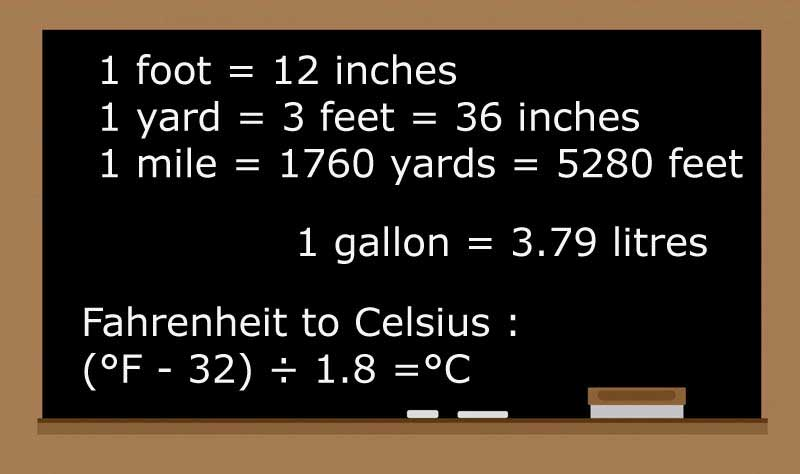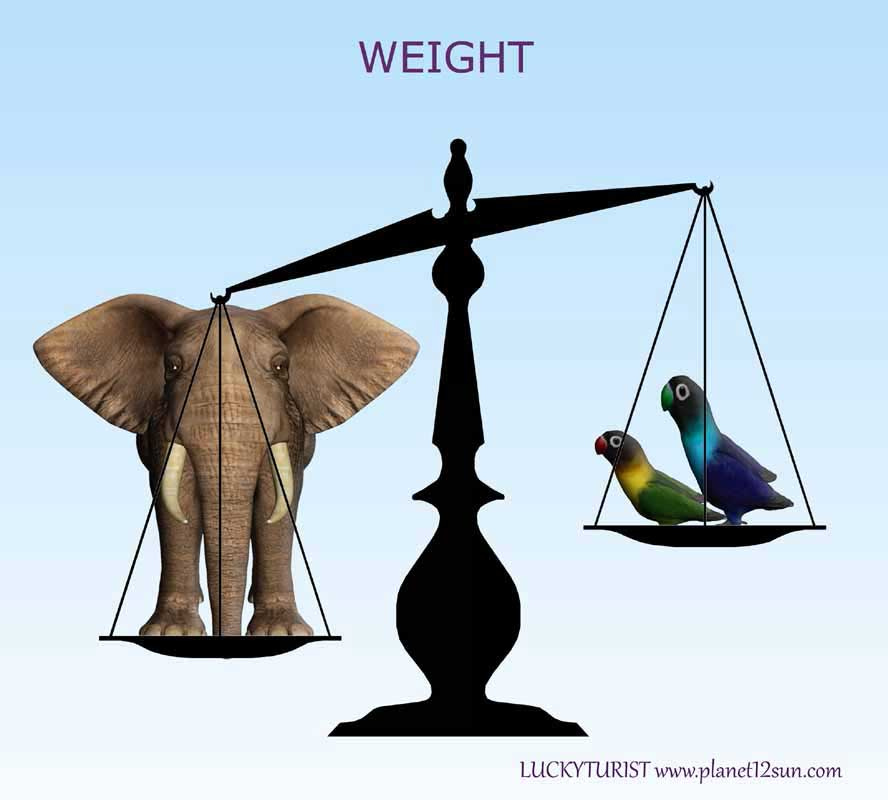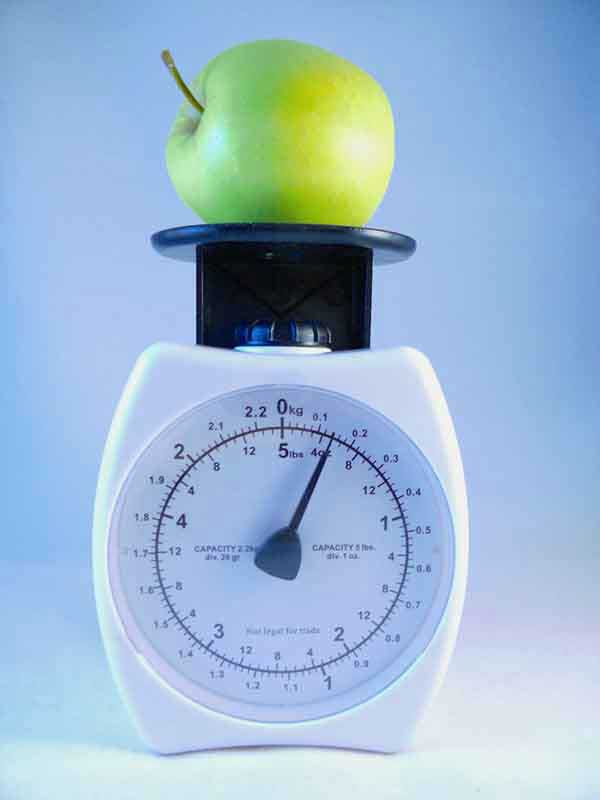## Weight & Mass

In science and engineering, the weight of an object is usually taken to be the force on the object due to gravity. For small objects on Earth, the weight force is directed towards the center of the planet. For larger objects, such as the Moon orbiting around the Earth, the force is directed towards the center of mass of the combined system. On the surface of the Earth, the weight is proportional to the mass. Objects which have the same mass have the same weight. (However, mass and weight are not the same thing).Units of Weight
The unit of weight in the International System of Units is the newton, which is represented by the symbol 'N'. For example, an object with a mass of one kilogram has a weight of about 9.8 newtons on the surface of the Earth, and about one-sixth as much on the Moon. In this sense of weight, a body can be weightless only if it is far away from any other mass.

In commercial and everyday use, the term "weight" is usually used to mean mass, and the verb "to weigh" means "to determine the mass of" or "to have a mass of". Used in this sense, the proper SI unit is the kilogram (kg). In United States customary units, the pound can be either a unit of force or a unit of mass.### Metric System

gram (g), kilogram (kg), tonne (t)

Gram The gram is the basic unit of weight and mass in the metric system. The definition of a gram was set to equal the mass of one cubic centimeter of water at maximum density. One gram is approximately 0.0022 pounds on the surface of the Earth.

Items commonly measured in grams: flour, bread, pasta, sugar, butter... A paperclip weighs about 1 gram.

Kilogram For larger, more massive items over 1,000 grams, a kilogram measurement is used. One kilogram equals 1,000 grams. Most scales use the metric system and report the weights of larger items, such as animals and humans, in kilograms. For example, a doctor weighs a patient in kilograms.

Tonne To measure things that are very heavy, we use tonnes (also called metric tons). 1 tonne equals 1,000 kilograms.

Items commonly measured in tonnes: cars, rock, cargo, dirt...### US Standard Units (English Units, US Customary Units)

ounce (oz), pound (lb), ton

Ounce The smallest unit of mass is ounces. If you mean an ounce of fluid (volume) say "fluid ounce" ("fl oz"), otherwise ounce usually means mass.

Items commonly measured in ounces: herbs, cooking spices, bread...

Pound One pound equals 16 ounces. The abbreviation is "lb", because it came from the Latin word "libra".

Items commonly measured in pounds: flour, fruits and vegetables, sugar, people, animals...

Ton 1 ton equals 2,000 pounds. Heavy things like cars, trucks, ships and heavy equipment are measured using tons instead of pounds. In some countries a "ton" can mean 2,240 pounds, so to be clear use "short" or "long" in front of "ton", like this:

- a short ton is 2,000 pounds
- a long ton is 2,240 pounds